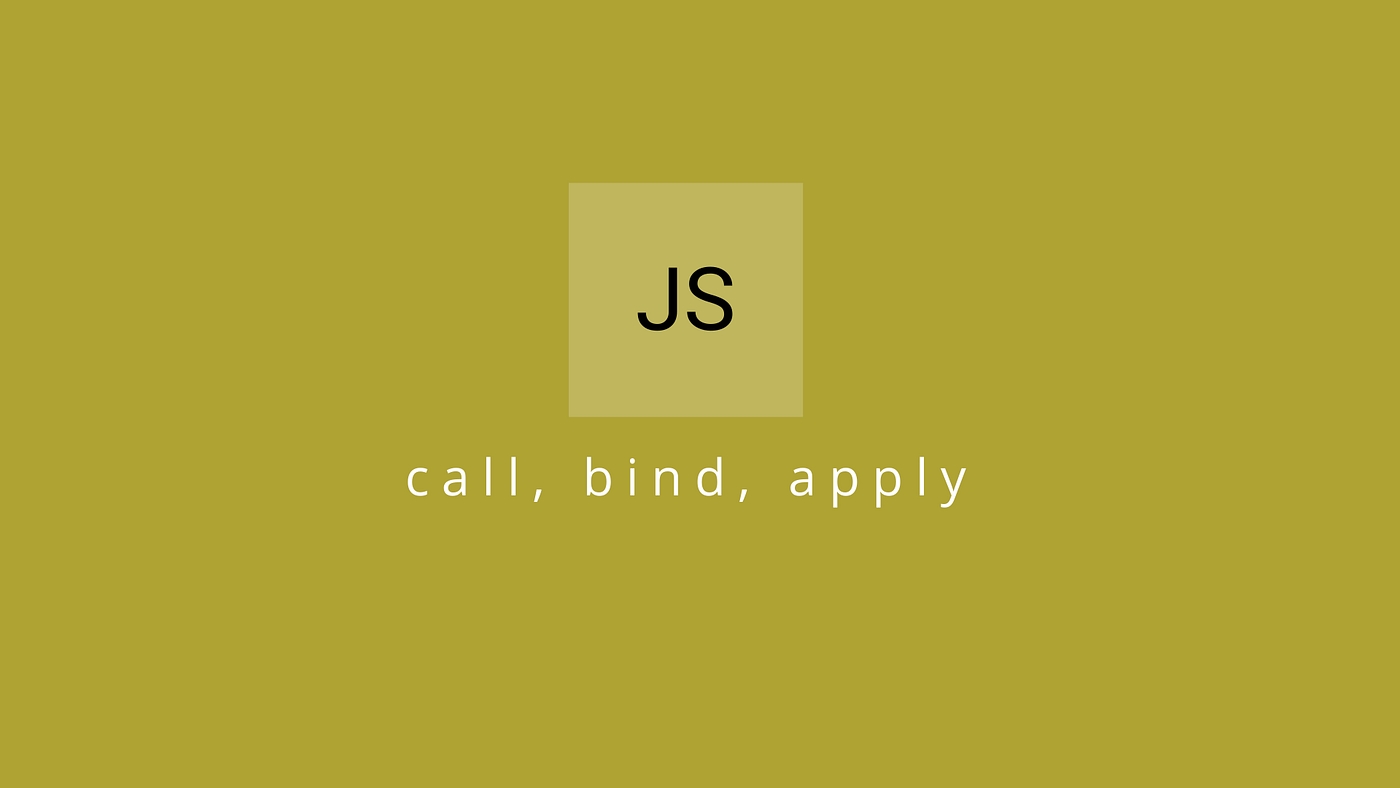# Call, Bind and Apply in JavascriptA - Apply | B - Bind | C - Call

J ust to make it more simple, These three work the same and are used for the same purpose i.e to maintain “this” keyword.
Okay, now we know that why they are used. But why are three methods require to serve one purpose, isn’t it weird? 🤔
This blog will help you so to know how and where these three methods are used. Chalo!!! let’s begin our blog.

Let’s take a simple module as an example

So, In the above example, It is very clear that the variable “caller” has access to a method in callModule right!!
So what will be the output if I call caller() directly.
Any Guesses!!! 😝
If the output is “Abhishek”, then there was no need for this blog 😆.
So the outcome is nothing gets printed in the console.

Now, the question is why? why?
let’s step back and analyse. See in the code the variable “caller” has only access to a method in the callModule but it doesn’t have any access to the data which is there in the module. So, to achieve this, we will need the help of these three methods. Now, let’s understand what is the significance of them.

# Call

As I already mentioned, the variable “caller” has access to only a method in the module but not the entire module. So let’s give the variable “caller” the reference of callModule.

Hence, the caller variable has the reference of callModule where it can access the data variable inside it and eventually, the outcome is the name gets printed.

Now, we know the significance of call but can I use the other two here?
Before answering this, let’s know about the other two first and then you can only answer this question.

# Apply

Let’s make it clear now, call and apply are exactly the same, the only thing which differentiates them is the way function parameters gets passed into them.
In call, Parameters are passed individually but in apply it gets passed as an array.

So, you get the same result which you got when you used call.

You got your answer both call and apply can be used depending on the function parameters. so let’s find if we can use bind for the same scenario or not 😃.

# Bind

As We know that JS is Synchronous but with the help of promises it can be made asynchronous.
https://medium.com/quinbay/event-loop-in-javascript-4fdc862d5fa7
promoting my own stuff 😝.

Yes, let me clear it, So think of a scenario where you need to call a specific function after successful execution of the other which is asynchronous.
Can we use call and apply in this scenario, let’s check that
Let’s take a dummy API call where we get some data and upon successful call, we need to call one function printing the response.
What actually bind does?
Bind will maintain this keyword right, It will have the reference of that module and but unlike Call and Apply it won’t execute the function as soon as it is assigned but bind will store reference of that Object and we need to call it explicitly whenever it is supposed to be called.

Now, You understand why I mentioned API calls here. If you want to call a function after the success of some other function then a bind will be used for it.
So, Let’s get back to our question Can we use it like Call() and Apply()
but we need to call the function explicitly after binding the Object Reference.

Now, We came to know what is Call(), Apply() and Bind(). Hope this blog helped you to understand better about the above methods.

To make it more interesting!!
Let’s guess the output of the given Snippet. Please give your answers in the comment section with the reason behind it.